# Sculpting to Meet Average Debt Life with Fixed Debt Size

This page addresses the debt sculpting in the case where the amount of debt is established from the debt to capital ratio and you have a term sheet with a defined minimum DSCR and the maximum debt life.  The sculpting challenge here is that you cannot use a couple of simple mathematical formulae to derive the repayment pattern as you can when the debt size is driven by prospective cash flow and by the DSCR. It is a big challenge. In this case where the debt size is driven by the debt to capital ratio, sculpting does not mean that the DSCR is constant but instead the is some kind of pattern driven by a non-constant DSCR that cannot violate some kind of minimum DSCR criteria.  When the DSCR is greater than the minimum in some periods, there is less debt service and dividends can be higher. The modelling in this case is a lot more complicated than the basic situation where the DSCR is constant. Where the debt to capital constraint drives debt size and a minimum DSCR is defined in a term sheet, the pattern of debt repayment depends in part on the average debt life. The manner in which returns are affected by different minimum DSCR, average life and debt to capital constraints with different cash flows is described using different scenarios. If you are negotiating a term sheet, the analysis described on this page will help you understand what terms are important and what terms do not make much of a difference.

## Files that Address the Issue of Computing Sculpting Repayments from Curved DSCR to Meet Average Life and Minimum DSCR Constraints

I have a few files that you can download by pressing the buttons below in order to address this sculpting issue. I have wasted a lot of time coming up with a user defined function that can developed curved sculpting in the case where the debt to capital is given and the minimum DSCR is also defined. I am in the process of refining the process, but you can download the current state of the model below.  Underneath the button for downloading the file with the UDF I have included a couple of screenshots from the file that computes the shape of DSCR that declines from LLCR multiplied by a positive factor and works down to the minimum DSCR. Note that the average debt life constrains things.  If the average debt live is near the minimum debt life you cannot skew the repayments to the end which also means you cannot curve the DSCR.

## Really Simple Example of Sculpting with Maximum Debt to Capital Constraint and Non-Constant DSCR

To illustrate the problem of sculpting with debt determined from the project cost multiplied by a debt to capital percent, consider an absurdly simple example. Assume four years of cash flow all of 100.  Assume also a maturity of 3 years and a minimum debt service coverage of 2.0.  Finally, assume that the project cost is 250, the maximum debt to capital is only 40% and the minimum allowed average debt life is 2.4 years.  The screenshot below displays these assumptions (in blue) and calculation of the debt size if the debt size were determined from the minimum DSCR as well as the projected cash flow. In this case the debt service is computed as 100/2 or 50 for each of the three years of the loan. The amount of the loan from the DSCR sizing would be 50 x 3 or 150 — this is using the fundamental rule the the NPV of the debt service is equal to the debt at commercial operation. The average debt life of the loan is the sum of the repayment (there is no interest, remember) multiplied by the year of the loan all divided by the amount of debt at commercial operation.  In this case the loan life is 1 x 50 + 2 x 50 + 3 x 50 or 300 divided by 150 or 2.0 years.  The example illustrates that the average loan life is the middle when the repayment is equal (debt service and repayment are the same as the interest is zero.)  Notice that the size of the loan is greater than the maximum loan allowed and that equity IRR is 43.7%.  The equity IRR is computed from the negative of project cost and debt followed by the cash flow of 100 minus the debt service of either 50 or zero.  We will see that we cannot obtain this level of IRR when the debt is constrained, but the sculpting will affect the equity IRR.The next screenshot moves to debt repayment in the case where debt is limited by the debt to capital ratio.  A repayment pattern can be used in the same way as for the repayment using the DSCR to size the debt, but with a different DSCR.  To compute the similar debt repayment, you can use the principle that the DSCR in each period is the same as the LLCR if sizing with the DSCR is applied.  As the LLCR is the present value of cash flow (over the debt term) divided by the debt, you can compute the LLCR from the debt given by the maximum debt to capital ratio, which in our simple example is 100 instead of 150.  The PV of the CFADS over the loan life is 300 because of our assumption that the interest rate is zero. Therefore the LLCR is 300/100 or 3.  You can apply this LLCR and compute the period by period debt service to be 100/3 = 33 as shown in the screenshot below.  Note that because of the lower amount of debt, the equity IRR has fallen from 43% to 32%. Note that in this example, the minimum and the average DSCR is 3 times rather than the minimum of 2.0 times that was the assumption in the construction of our very simple example.  Finally, note that the average debt life — the sum of the repayment and the debt year divided by the debt balance is 2.0 year in this example, which is the same as in the first example.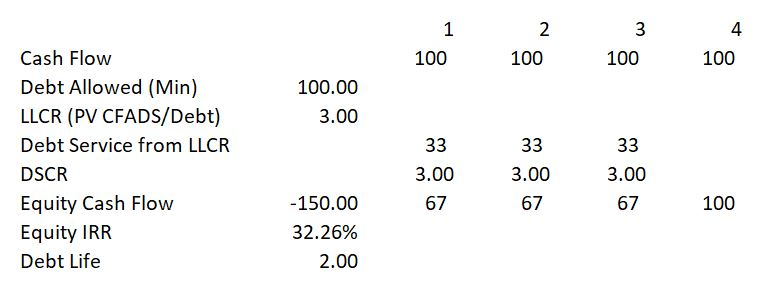The above example does not really reflect the term sheet because the term sheet allows the minimum DSCR to decline to 2.0.  So you could change the repayment patterns around and make the DSCR 2.0 instead of 3.0 which was the DSCR in LLCR case. In the screenshot below I have manually set the debt service to be 50 in the second year and 50 in the third year.  This produces a DSCR of 2.0 times in the third and the fourth year which conforms to the requirement in the assumption.  In the first year there is no debt service and a higher dividend is allowed.  When the debt service is pushed back in this way, the equity IRR is greater than if the debt service were computed from the case where the debt service is driven by the LLCR.  With the debt service pushed back, the DSCR is infinite in the first period and the equity IRR has increased from 32.26% to 35.85%. But note that in this case the average loan life has increased to 2.5 which does not conform to the requirement in the assumption section, which was 2.4 years.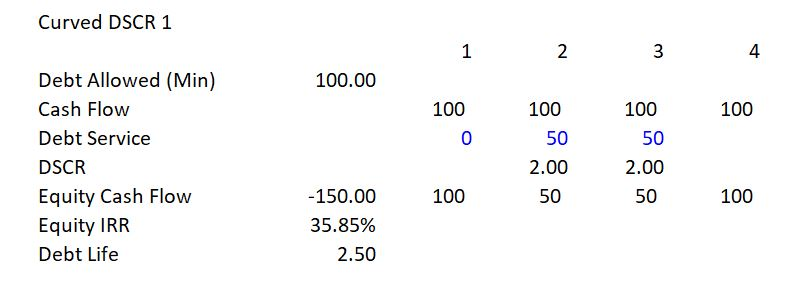You could come up with an infinite number of ways to change the debt service for the three years and still come up with the total required debt service of 100 over the debt term.  One thing that you would not want to do is to push the debt service too early.  This is shown in the screenshot below where the debt service is 50 in the first two years and nothing in the third year.  It may be obvious to you that you would not want to do this, but I am presenting the example to illustrate what happens when the average life is shorter than the required life.  In this case, the minimum DSCR constraint is met and the average loan life is shorter than the specified average loan life, meaning the debt life constraint is also met. But because the dividend is pushed back later to the third year instead of being pushed forward to the first year as in the prior example, the equity IRR is reduced.  Whereas the equity IRR was 35.85% in the case with the delayed debt service, the equity IRR is now lower than the LLCR case at a level of 29.51%.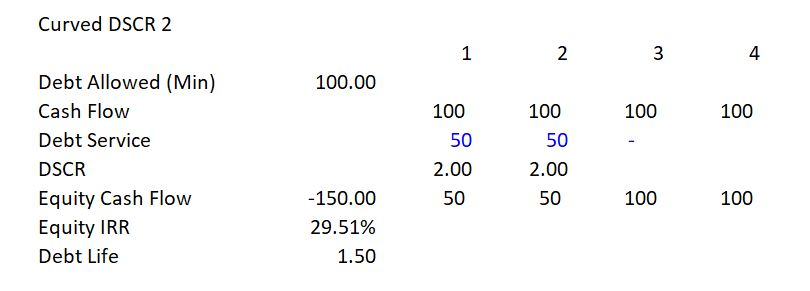In the next case illustrated on the screenshot below, I have manually adjusted the debt service to include 10 of repayment in the first year; 40 of repayment in the second year; and 50 of repayment in the third year.  This repayment pattern accomplishes three objectives as illustrated in the screenshot. First, the total amount of debt is repaid. Second, the minimum DSCR constraint is met as the lowest DSCR occurs in the third period and it is 2.0 while the DSCR’s in second period and the third period are higher — 10 in period 1 and 2.5 in period 2. Third, as shown on the bottom of the screenshot, the debt life is 2.40 which just hits the constraint in the assumptions that were presented at the beginning of this section.  With this debt repayment, the equity IRR is 35.01% which is quite a lot higher than the 32.26% equity IRR that was hit using the LLCR method where the DSCR was constant but never hit the minimum.In the last three cases above I input the debt repayment directly.  The last question I address in this section is whether you can come up with a method to compute the optimal sculpting that is shown in the final screen shot where the debt life reached 2.40.

## Sculpting Theory and Sample Term Sheet

In this section I illustrate how the kind of assumptions that I presented in the simple example can be reflected in term sheets or at least in negotiations between a developer and a sponsor. I present three excerpts from a term sheet where the debt to capital constraint is defined; the minimum DSCR is specified; and, the average loan life limit is specified. The excerpt from a term sheet shown below illustrates the point that in a term sheet that is being negotiated, the debt to capital ratio is a maximum constraint and the debt could be lower if the DSCR constraint results in a lower amount of debt. For the purpose of this page, I assume that the maximum debt to capital constraint is in place.  Further discussion of the debt to capital constraint versus the DSCR constraint is discussed on another page of my website that is linked to this sentence.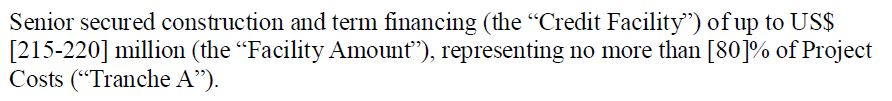The sculpting methods in other pages that address sculpting when there is a debt to capital constraint use what I refer to as the LLCR method to sculpt debt.  For example, assume the minimum DSCR is 1.35 and the maximum debt to capital ratio is 80%. Say also that the debt to capital constraint is in place, meaning that if you sculpted to achieve the minimum DSCR in each period, the debt to capital would exceed 80%. The LLCR method works by changing the target DSCR in the sculpting equation. This means that if the debt to capital constraint is limiting the debt size and then, you could increase the DSCR so as to achieve the debt to capital constraint. You can do this with the LLCR formula which is nice an elegant, but it generally will not appropriately model the structuring issues. If the DSCR is allowed to fall to the minimum level over the life of the project the DSCR does not have to be constant.  If the DSCR is allowed to be higher at the beginning of the debt life and then fall to the minimum level, then the payments in the early part of the life of the project are reduced. This means that a level or flat DSCR over the debt life at a higher level than the LLCR computed flat amount is optimal. This idea is demonstrated in a simple example below with screenshots.

The first screen shot shows various components of a term sheet. Note that the minimum DSCR is specified as well as the maximum debt to capital. In addition, the minimum average debt life is stated as a constraint.  In this term sheet, if the DSCR of 1.35 results in a debt to capital ratio of above 80%, then the debt to capital constraint will be in place.  The minimum and average DSCR from tailoring the debt are not specified, but it is possible that the minimum DSCR can be 1.35 and the average DSCR can be above 1.35 (like the LLCR).  In this case, the average debt life of 10 must be maintained. Note first the language about the DSCR language. The minimum and average cannot be below 1.35. But the minimum could be 1.35 and the average could be above 1.35.  This could be the case if the LLCR sculpting is above 1.35 as shown a little bit later: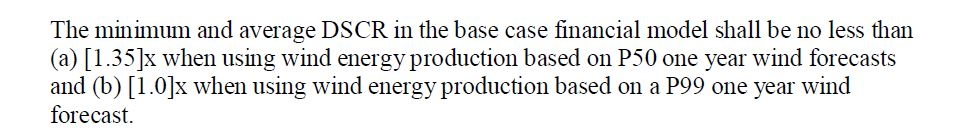Note the how the maturity provision works.  If you mess around with the DSCR, the average life of the loan must still not exceed 10 years.  This means that you could have changing DSCR’s all over the place, but the minimum must still be 1.35.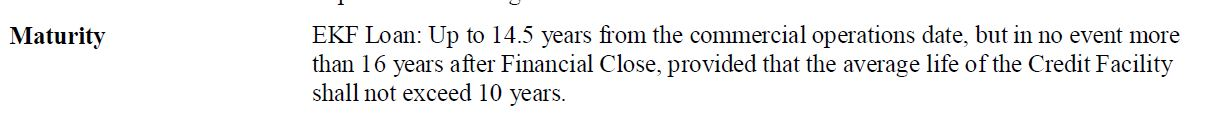So, let’s take a case where the DSCR is 1.35, but if you use 1.35 in every year, then the debt to capital will be too high.  This applies the rule that the NPV of debt is from the DSCR and CFADS.  By constraining the debt, you could come up with the scenario below with where the LLCR is used as the target in sculpting.

1. Sumproduct method: Multiply the period of the debt by the repayment for each period. Then sum the product and finally divide the product by the opening balance of the debt. This demonstrates that the average loan life is indeed the weighted average life of the repayments.

2. Opening Balance method: Sum the opening balance of the debt (it gets smaller as the debt is repaid). Divide the sum of the opening balance by the balance at COD.

In the case without debt constraint (where the debt is sized by the DSCR and not the debt to capital ratio), you can demonstrate the effect of the DSCR and the interest rate on the average loan life.  This is demonstrated in a data table below.

A Rule of thumb for max Average loan life is 0.7x of the door-to-door tenor, or .7 x 16 = 11.2, or  7 x 14.5 = 10.15.

Instead, you can design the sculpting so that the minimum DSCR will still be obtained, but that: (1) the total debt will be repaid (NPV of debt service) = debt; and (2) the average loan life is less than a specified loan life in the term sheet or loan agreement.

## UDF and Interpolate to Derive the Sculpted Repayment Pattern

In this section I discuss how to perform the process much faster and more efficiently with a UDF function.  The big deal about this is coming up with a very efficient process for the dynamic goal seek.  So, this paragraph introduces the UDF that is a central part of analysis of advanced sculpting.  To make the process work, you should first put the interpolate lookup function into your excel file and make a little table with time and a DSCR that changes.  This little table allows for all sorts of different “artistic” or “sculpted” shapes for the DSCR.  You can change the starting DSCR that puts different slopes into the model.  You can also change the ending time so that you can have different flat parts at the end.  The table for “designing” a sculpted shape is shown in the screenshot below.  The table is on the left at the top.  You need to come up with the method to change the starting DSCR and then you can make a data table with different end points.  The end time is 4 so the curve flattens out at the 1.10 minimum.  The start point is just above 6.0 as shown on the graph.  Finally, you can see that the closing balance of the debt and see that it is zero.  The parameters in the little table have to be set with a goal seek so that the the DSCR’s are at the correct level to repay the debt.  As explained with the simple example, you can make a data table to show what happens when the end date changes.  Note that is only one shape that works to repay the debt when you change the end time in the little square.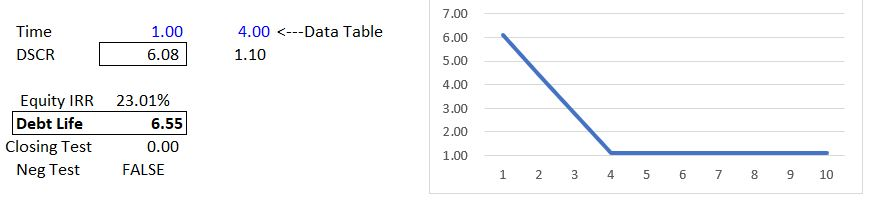The big deal is to come up with an efficient way to make the goal seek work really fast and does not blow up.  First, think about changing the end time to 1.5 so that the DSCR must jump down to the minimum almost immediately. This is probably impossible and the goal seek tool in excel will blow up.  You can avoid this by making a dynamic goal seek.  The dynamic goal seek works with a slope function and can be programmed to make sure it does not blow up (I assume just about everybody knows what I mean by the goal seek blowing up).  So, you are trying to set the total amount of the debt to zero, by changing the initial DSCR level.  This initial DSCR level is in turn is computed by a fixed number, multiplied by the LLCR (remember, the LLCR is the PV of CFADS divided by the fixed level of debt — both parameters are independent of the DSCR and sculpting).  The code for the dynamic goal seek is shown in the excerpt below.

.

```Function llcr_factor_gs()

Dim output1(1, 20) As Single

' First iterate around the LLCR factor to find close

llcr_increment = (max_llcr - 1) / 20
Count = 1
iter_count = 0

linear_interpolate = True
pv_debt_service = 0
net_pv = 0
debt_balance = debt_amount
Start_factor = 1
end_factor = max_llcr

re_start:

iter_count = iter_count + 1

If iter_count > 5 Then GoTo slope_intercept:

For llcr_fac = Start_factor To end_factor Step llcr_increment

Count = Count + 1

last_pv = net_pv

last_llcr_fac = llcr_fac - llcr_increment

period_range(1) = 1
period_range(2) = end_time
dscr_range(1) = llcr * llcr_fac
dscr_range(2) = min_dscr
compound_factor = 1
pv_debt_service = 0
debt_balance = debt_amount

For i = 1 To tenure

interest_expense(i) = debt_balance * interest_rate(i)
debt_bal(i) = debt_balance

If linear_interpolate = False Then dscr(i) = interpolate_look_up1(i, period_range, dscr_range)
If linear_interpolate = True Then dscr(i) = interpolate_look_up_linear1(i, period_range, dscr_range)

ebit = ebitda(i) - dep_exp(i)
ebt = ebit - interest_expense(i)

taxes(i) = ebt * tax_rate

If dscr(i) <> 0 Then debt_service(i) = cfads(i) / dscr(i) - other_debt_service(i) ' Now compute the debt service as pv of cfads
'
repayment(i) = debt_service(i) - interest_expense(i)

debt_balance = debt_balance - repayment(i)

compound_factor = compound_factor * (1 + interest_rate(i)) ' Overall debt IRR
pv_debt_service = pv_debt_service + debt_service(i) / compound_factor ' PV of debt service is debt

Next i

net_pv = debt_amount - pv_debt_service
If net_pv > 0 Then Exit For

Next llcr_fac

Start_factor = llcr_fac - llcr_increment
end_factor = llcr_fac + llcr_increment
llcr_increment = llcr_increment / 10

GoTo re_start:

slope_intercept:

'
' Now try slope and intercept
' Slope is pv_debt_service/llcr_factor
'
If (llcr_fac - last_llcr_fac) <> 0 Then _
Slope = (net_pv - last_pv) / (llcr_fac - last_llcr_fac)

Intercept = net_pv - Slope * llcr_fac

If Slope <> 0 Then llcr_fac_final = -Intercept / Slope

output1(1, 1) = llcr_fac_final

llcr_factor_gs = output1

End Function

.

.```

You need to read a bunch of stuff into the UDF.  This is accomplished with a table and a program that reads in the data.  You can see a picture of the variables that you need to read into the UDF in the screenshot below.  I have made this so you can be flexible where you put the data and you can use the entire line (as I complain about the using the LOOKUP function).  I put the code you can use to read in the data from a table (not a data table, please do not get confused).  In the screenshot, the data that is read into the UDF is shown in the box.  When you look at the box you can see that some of the variables are single numbers — scalars.  Other numbers, starting with the EBITDA are time series.  The UDF shows how you can read in the different types of variables and how I have put in separate definitions and worked with the arrays.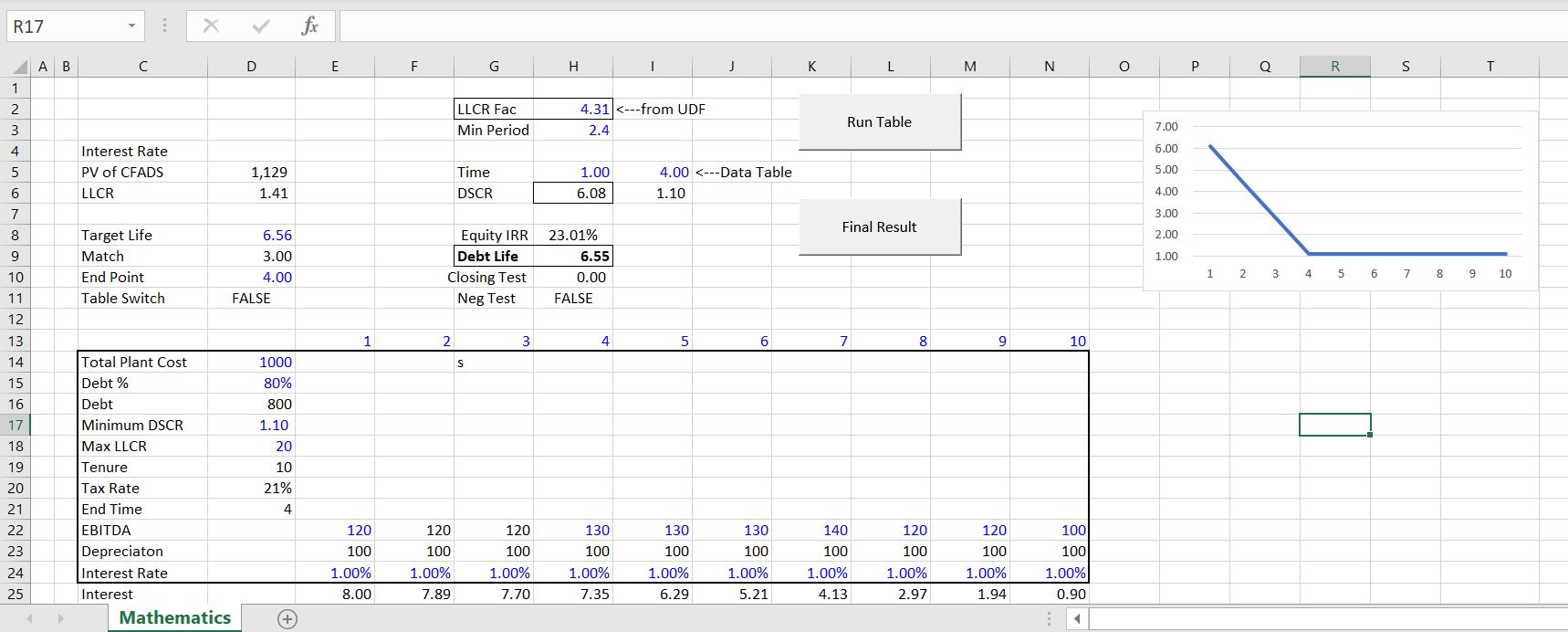.

```Function operation_read(operational_inputs As Variant) As Variant ' operational_inputs defined here

operation_row = operational_inputs.Rows.Count
operation_cols = operational_inputs.Columns.Count

count1 = 0
count2 = 0
count3 = 0

For i = operation_row To 1 Step -1 ' first loop around the rows with i counter. Start at end and work backwards

For j = 1 To 500 ' loop around the colums with j counter

If i = 1 Then ' reading array: Standby Interest Rate
If WorksheetFunction.IsNumber(operational_inputs(i, j)) = True Then ' When found the first row
cost = operational_inputs(i, j) ' Assign to fixed when found a row
Exit For ' DO NOT exit loop, but go to the next column
End If
End If

If i = 2 Then ' reading array: Standby Interest Rate
If WorksheetFunction.IsNumber(operational_inputs(i, j)) = True Then ' When found the first row
debt_pct = operational_inputs(i, j) ' Assign to fixed when found a row
Exit For ' DO NOT exit loop, but go to the next column
End If
End If

If i = 3 Then ' reading array: Standby Interest Rate
If WorksheetFunction.IsNumber(operational_inputs(i, j)) = True Then ' When found the first row
debt_amount = operational_inputs(i, j) ' Assign to fixed when found a row
Exit For ' DO NOT exit loop, but go to the next column
End If
End If

If i = 4 Then ' reading array: Standby Interest Rate
If WorksheetFunction.IsNumber(operational_inputs(i, j)) = True Then ' When found the first row
min_dscr = operational_inputs(i, j) ' Assign to fixed when found a row
Exit For ' DO NOT exit loop, but go to the next column
End If
End If

If i = 5 Then ' reading array: Standby Interest Rate
If WorksheetFunction.IsNumber(operational_inputs(i, j)) = True Then ' When found the first row
max_llcr = operational_inputs(i, j) ' Assign to fixed when found a row
Exit For ' DO NOT exit loop, but go to the next column
End If
End If

If i = 6 Then ' reading array: Standby Interest Rate
If WorksheetFunction.IsNumber(operational_inputs(i, j)) = True Then ' When found the first row
tenure = operational_inputs(i, j) ' Assign to fixed when found a row
Exit For ' DO NOT exit loop, but go to the next column
End If
End If

If i = 7 Then ' reading array: Standby Interest Rate
If WorksheetFunction.IsNumber(operational_inputs(i, j)) = True Then ' When found the first row
tax_rate = operational_inputs(i, j) ' Assign to fixed when found a row
Exit For ' DO NOT exit loop, but go to the next column
End If
End If

If i = 8 Then ' reading array: Standby Interest Rate
If WorksheetFunction.IsNumber(operational_inputs(i, j)) = True Then ' When found the first row
end_time = operational_inputs(i, j) ' Assign to fixed when found a row
Exit For ' DO NOT exit loop, but go to the next column
End If
End If

If i = 9 Then
If WorksheetFunction.IsNumber(operational_inputs(i, j)) = True Then ' When found the first row
count1 = count1 + 1
ebitda(count1) = operational_inputs(i, j)
End If
End If

If i = 10 Then
If WorksheetFunction.IsNumber(operational_inputs(i, j)) = True Then ' When found the first row
count2 = count2 + 1
dep_exp(count2) = operational_inputs(i, j)
End If
End If

If i = 11 Then
If WorksheetFunction.IsNumber(operational_inputs(i, j)) = True Then ' When found the first row
count3 = count3 + 1
interest_rate(count3) = operational_inputs(i, j)
End If
End If

Next j ' end of loop around colums in each row of the table -- often use exit for to go to next row

Next i ' end of loop around variables (rows in the table)

end_of_debug_operation: ' this is for skipping the debug routine

End Function

```

.

Once you have the goal seek defined, you can run the data table.  When you run the data table, it has to re-compute the starting DSCR with the LLCR factor.  The outputs of the UDF function with the goal seek and the code for the data table are shown below along with a couple of screenshots. The screenshots show how you make a little financial model and how you get the various outputs from the UDF.Here is the code for making the data table and the code for the UDF other than the goal seek.

.

```Function average_debt_life_table(operational_inputs, Optional table_switch) As Variant

Dim output(40, 40) As Double

a = llcr_compute()

If table_switch = False Then a = llcr_factor_gs()

If table_switch = 10 Then

For end_point = 2 To 20 Step 0.25

end_time = end_point

a = llcr_factor_gs()

If Abs(Range("closing_test")) > 2 Then Cells(Row, 18) = 10

Next end_point
End If

' Find the min with function

output(1, 1) = llcr_fac
output(2, 1) = cost
' output(2, 1) = debt_pct
output(3, 1) = debt_amount
output(4, 1) = min_dscr
output(5, 1) = max_llcr
output(6, 1) = tenure
output(7, 1) = tax_rate
output(8, 1) = end_time

For i = 1 To 20
output(9, i) = ebitda(i)
output(10, i) = dep_exp(i)
output(11, i) = taxes(i)
Next i

output(13, 1) = llcr

For i = 1 To 20
output(14, i) = debt_service(i)
output(15, i) = repayment(i)
output(16, i) = interest_expense(i)
output(17, i) = dscr(i)
output(18, i) = interest_rate(i)
Next i

output(19, 1) = pv_debt_service

For i = 1 To 20
output(21, i) = debt_bal(i)
If debt_amount <> 0 Then repayment_percent(i) = repayment(i) / debt_amount
output(22, i) = repayment_percent(i)
Next i

average_debt_life_table = output

End Function

Function llcr_compute()

Dim output1(1, 20) As Single

' First iterate around the LLCR factor to find close

llcr = min_dscr

For iter = 1 To 3

debt_balance = debt_amount

pv_debt_service = 0
compound_factor = 1

For i = 1 To tenure

interest_expense(i) = debt_balance * interest_rate(i)
debt_bal(i) = debt_balance

ebit = ebitda(i) - dep_exp(i)
ebt = ebit - interest_expense(i)

taxes(i) = ebt * tax_rate

If llcr <> 0 Then debt_service(i) = cfads(i) / llcr - other_debt_service(i) ' Now compute the debt service as pv of cfads
'
repayment(i) = debt_service(i) - interest_expense(i)

debt_balance = debt_balance - repayment(i)

compound_factor = compound_factor * (1 + interest_rate(i)) ' Overall debt IRR

pv_debt_service = pv_debt_service + debt_service(i) / compound_factor ' PV of debt service is debt

Next i

If debt_amount <> 0 Then llcr = pv_cfads / debt_amount

Next iter

End Function

.

```
```Sub Button25_Click()

Row = 52

For end_point = 2 To 20

Row = Row + 1

Range("period") = end_point

'llcr_fac = average_debt_life_table(Range("inputs"))

' llcr_fac = WorksheetFunction.Sum(Range("inputs"))

Cells(Row, 16) = end_point

Cells(Row, 17) = Range("irr")
Cells(Row, 18) = Range("computed_life")
Cells(Row, 19) = Range("closing_test")
Cells(Row, 20) = Range("neg_test")

If Abs(Range("closing_test")) > 2 Then Cells(Row, 18) = 10

' MsgBox "llcr_fac" & llcr_fac

Next end_point

End Sub```

## Illustrative Case Study of Sculpting, Constraints and Equity IRR

The file and screenshots below are designed to provide an understand the negotiating implications of parameters that affect IRR with advanced sculpting.  The sculpting is designed to produce a varying DSCR that is targeted to achieve constraints of both: (1) a target debt life; and, (2) a minimum DSCR.

The advanced sculpting is compared to two other cases.  The first alternative case is one where there is no curvature in the DSCR.  The second is the case where there is not debt to capital constraint and the debt size is driven by the minimum DSCR in each year.  An excel model that includes these three cases and is used for the exercises is available for download by pressing the button below.

This section will demonstrate that the average life of a credit facility can have a big effect on the ultimate equity IRR for the investor.

If the average debt life is relatively short, the minimum DSCR in the term sheet may not be obtainable because of the constraint that the debt size is driven by the repayments.  On the other hand, if the minimum DSCR is low, a long debt life may allow initial repayments to be reduced and a higher IRR to be obtained.

To illustrate the process, you should understand how the average debt life works.  If the repayment occurs at the end of the period which is the standard approach you generally use in analysis and modelling, then the average loan life can be computed in two ways as follows:

1. Sumproduct method: Multiply the period of the debt by the repayment for each period. Then sum the product and finally divide the product by the opening balance of the debt. This demonstrates that the average loan life is indeed the weighted average life of the repayments.

2. Opening Balance method: Sum the opening balance of the debt (it gets smaller as the debt is repaid). Divide the sum of the opening balance by the balance at COD.

In the case without debt constraint (where the debt is sized by the DSCR and not the debt to capital ratio), you can demonstrate the effect of the DSCR and the interest rate on the average loan life.  This is demonstrated in a data table below.

(If you don’t know what this means, you need to review the debt to capital versus the DSCR sizing analysis in the advanced structuring section.) As the average life drives the pattern of repayment, this section will demonstrate that the average life of a credit facility can have a big effect on the ultimate equity IRR for the investor.

To illustrate this case, I begin with a case where the debt to capital constraint is in place (because of a high debt life, a long debt tenure or a low interest rate).

## No “Go Pro” Here: If you want to be Really good, there is no alternative but to sign up for an In-Person Course

The reason I have worked on this website is so that you consider an in-person class which is by far the best way you can become a top project finance analyst. I could try and create some kind of half baked model that is not really complete and then beg you to pay for a complete model (this is like go pro products in wordpress).  What B.S.  The only way to use the advanced models is to learn how to modify them and how to implement them in your situations.  You need to attend a course for this. If you click on the button below, you will be forwarded to a website that describes unique courses where you can learn about UDF’s, circular references, project finance structuring theory and creating a truly flexible model.

If you click on the right button you can quickly send an e-mail to edwardbodmer@gmail.com and request the resource library (no charge).  The google drives include more case studies, financial models, risk analysis files and other materials than are included on the website. I promise not to pester you if you do send me an e-mail.

I would really want to teach you some of the advanced issues and not the same old blah, blah, blah with fancy colours and masks etc. If we can get about three people together we can run the course. I would like to know where you would like the courses to be held. If you click on the left button below, I have a form that I will use to try and put together a class with a few people.